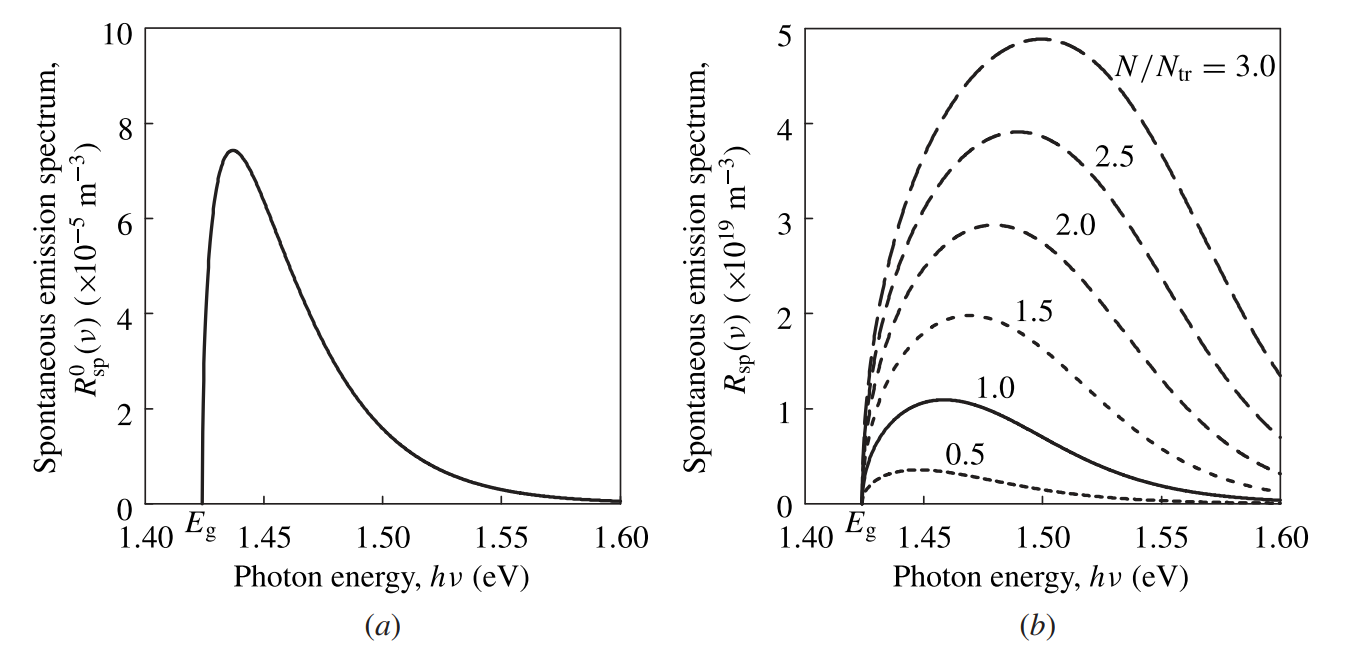# Spontaneous Emission in Semiconductors

This is a continuation from the previous tutorial - optical gain in semiconductors.

The spontaneous emission spectrum of a semiconductor can be explicitly related to the absorption and gain spectra of the semiconductor. By using (13-32) [refer to the optical gain in semiconductors tutorial] to eliminate $$\rho(\nu)$$ in (13-28) [refer to the band-to-band optical transitions in semiconductors tutorial], we find

$\tag{13-41}R_\text{sp}(\nu)=\frac{8\pi{n}^2\nu^2}{c^2}\alpha_0(\nu)f_\text{c}(E_2)[1-f_\text{v}(E_1)]$

Using (13-33), (13-34) [refer to the optical gain in semiconductors tutorial], and (13-41), we can express the spontaneous emission spectrum $$R_\text{sp}(\nu)$$ in terms of the absorption spectrum $$\alpha(\nu)$$ and the gain spectrum $$g(\nu)$$ as follows:

$\tag{13-42}R_\text{sp}(\nu)=\frac{8\pi{n}^2\nu^2}{c^2}\frac{\alpha(\nu)}{\text{e}^{(h\nu-\Delta{E}_\text{F})/k_\text{B}T}-1}=\frac{8\pi{n}^2\nu^2}{c^2}\frac{g(\nu)}{1-\text{e}^{(h\nu-\Delta{E}_\text{F})/k_\text{B}T}}$

In the case when $$N\lt{N}_\text{tr}$$, there is no optical gain but only absorption. In this situation, $$\alpha(\nu)\gt0$$ and $$R_\text{sp}(\nu)$$ has positive values for photon energies larger than the bandgap because $$h\nu\gt{E}_\text{g}\gt\Delta{E}_\text{F}$$ when $$N\lt{N}_\text{tr}$$.

In the case when $$N\gt{N}_\text{tr}$$, the semiconductor has an optical gain with $$g(\nu)\gt0$$ and $$\Delta{E}_\text{F}\gt{h\nu}\gt{E}_\text{g}$$. From (13-42), we find that $$R_\text{sp}(\nu)$$ again has positive values.

Therefore, as should be intuitively expected, $$R_\text{sp}(\nu)\ge0$$ for all frequencies no matter whether the semiconductor has a positive gain or not. Figure 13-9 shows the spontaneous emission spectra of GaAs (a) in thermal equilibrium and (b) at various levels of excess carrier density.Figure 13-9.  Spontaneous emission spectra of GaAs (a) in thermal equilibrium and (b) at various levels of normalized excess carrier density $$N/N_\text{tr}$$. Note that there is a difference of 24 orders of magnitude between the vertical scales of (a) and (b). These spectra are to be compared with the gain and absorption spectra shown in Figure 13-7 (a) [refer to the optical gain in semiconductors tutorial].

For a semiconductor in thermal equilibrium, $$\Delta{E}_\text{F}=0$$ and $$\alpha(\nu)=\alpha_0(\nu)$$. Then, its spontaneous emission spectrum is given by

$\tag{13-43}R_\text{sp}^0(\nu)=\frac{8\pi{n}^2\nu^2}{c^2}\frac{\alpha_0(\nu)}{\text{e}^{h\nu/k_\text{B}T}-1}$

This relation is known as the van Roosbroeck-Shockley relation.

Though we have obtained this relation by considering band-to-band transitions, it is a general relation that can be obtained by considering the equilibrium between the spontaneous emission of the semiconductor and the absorption of the surrounding blackbody radiation by the semiconductor. Consequently, it is generally valid for any transitions that contribute to $$\alpha_0(\nu)$$.

If we consider only band-to-band transitions, then (13-32) [refer to the optical gain in semiconductors tutorial] can be used to obtain

$\tag{13-44}R_\text{sp}^0(\nu)=\frac{8\pi{n}^2\nu^2}{c^2}\frac{\alpha_0(\nu)}{\text{e}^{h\nu/k_\text{B}T}-1}=\frac{1}{\tau_\text{sp}}\frac{\rho(\nu)}{\text{e}^{h\nu/k_\text{B}T}-1}$

Thus, the total band-to-band spontaneous recombination rate of electron-hole pairs in the thermal equilibrium state is

\tag{13-45}\begin{align}R_\text{sp}^0=\displaystyle\int\limits_0^\infty{R}_\text{sp}^0(\nu)\text{d}\nu&=\frac{8\pi{n}^2}{c^2}\int\limits_0^\infty\frac{\nu^2\alpha_0(\nu)}{\text{e}^{h\nu/k_\text{B}T}-1}\text{d}\nu\\&\approx\frac{2}{\tau_\text{sp}}\left(\frac{2\pi{m}_\text{r}^*k_\text{B}T}{h^2}\right)^{3/2}\text{e}^{-E_\text{g}/k_\text{B}T}\end{align}

where the functional form of $$\rho(\nu)$$ given in (13-17) [refer to the band-to-band optical transitions in semiconductors tutorial] is used in carrying out the last integration and the fact that $$E_\text{g}\gg{k}_\text{B}T$$ for most semiconductors of interest at room temperature is taken.

Accordingly, we find that $$\tau_\text{sp}$$ is related to the absorption coefficient $$\alpha_0(\nu)$$ by

$\tag{13-46}\frac{1}{\tau_\text{sp}}=\frac{4\pi{n}^2}{c^2}\left(\frac{2\pi{m}_\text{r}^*k_\text{B}T}{h^2}\right)^{-3/2}\text{e}^{E_\text{g}/k_\text{B}T}\displaystyle\int\limits_0^\infty\frac{\nu^2\alpha_0(\nu)}{\text{e}^{h\nu/k_\text{B}T}-1}\text{d}\nu$

This relation is analogous to that given in (10-45) [refer to the optical absorption and amplification tutorial] for atomic transitions.

In thermal equilibrium, the total recombination rate has to be balanced by the total generation rate. Therefore, if only bimolecular band-to-band transitions are considered, we also have

$\tag{13-47}R_\text{sp}^0=G_0=Bn_0p_0$

In a nondegenerate semiconductor where the law of mass action given in (12-31) [refer to the electron and hole concentrations in semiconductors tutorial] is valid, we can use (13-45) and (13-47) to express the bimolecular recombination coefficient $$B$$ in terms of $$\tau_\text{sp}$$ as follows:

$\tag{13-48}B=\frac{1}{2\tau_\text{sp}}\left(\frac{2\pi{k}_\text{B}T}{h^2}\right)^{-3/2}(m_\text{e}^*+m_\text{h}^*)^{-3/2}$

We have mentioned in the band-to-band optical transitions in semiconductors tutorial that $$\tau_\text{sp}$$ is not the same as either $$\tau_\text{rad}$$ or $$\tau_\text{s}$$. The fact can easily be seen by comparing (13-48) with (13-6) [refer to the radiative recombination in semiconductors tutorial].

Indeed, both $$\tau_\text{rad}$$ and $$\tau_\text{s}$$ are functions of the excess carrier density $$N$$, but $$\tau_\text{sp}$$ is an intrinsic parameter of a semiconductor that is independent of the excess carrier density.

Clearly from (13-46) and (13-48), the values of both $$\tau_\text{sp}$$ and $$B$$ vary significantly with temperature. In practice, they also depend on other mundane conditions, such as the impurities and defects in a sample.

In the case of GaAs at $$300\text{ K}$$, for example, the relation from (13-46) yields a theroretical value of $$\tau_\text{sp}\approx500\text{ ps}$$, corresponding to $$B\approx1.8\times10^{-16}\text{ m}^3\text{ s}^{-1}$$.

Experimentally measured values for the $$B$$ coefficient of GaAs are typically smaller than the theoretical value; they fall in the range of $$0.5$$ to $$2\times10^{-16}\text{ m}^3\text{ s}^{-1}$$ at room temperature, for corresponding values of $$\tau_\text{sp}$$ in the range between 2 ns and 500 ps.

In contrast, $$\tau_\text{rad}$$ can be anywhere from 500 ps to the order of seconds, depending on the excess carrier density and the amount of impurities in the material.

From these discussions, it can be clearly seen that $$\tau_\text{sp}$$ is a parameter that reflects the strength of the coupling between the electrons making optical transitions and the optical radiation emitted or absorbed by electrons. The smaller the value of $$\tau_\text{sp}$$, the stronger the coupling is and the more efficient the interaction between the electrons and the radiation is.

Example 13-5

Calculate the value of the total spontaneous emission rate $$R_\text{sp}^0$$ and that of the bimolecular recombination coefficient $$B$$ for GaAs at $$300\text{ K}$$ with $$\tau_\text{sp}=500\text{ ps}$$.

From Example 13-2(a) [refer to the band-to-band optical transitions in semiconductors tutorial], we know that $$m_\text{r}^*=0.0594m_0$$ for GaAs. At $$300\text{ K}$$, $$E_\text{g}=1.424\text{ eV}$$ for GaAs and $$k_\text{B}T=25.9\text{ meV}=0.0259\text{ eV}$$.

Using (13-45), the total spontaneous emission rate can be calculated:

\begin{align}R_\text{sp}^0&=\frac{2}{500\times10^{-12}}\times\left[\frac{2\pi\times0.0594\times9.11\times10^{-31}\times0.0259\times1.6\times10^{-19}}{(6.626\times10^{-34})^2}\right]^{3/2}\times\text{e}^{-1.424/0.0259}\text{ m}^{-3}\text{ s}^{-1}\\&=9.64\times10^8\text{ m}^{-3}\text{ s}^{-1}\end{align}

Using (13-48) with $$m_\text{e}^*=0.067m_0$$ and $$m_\text{h}^*=0.52m_0$$ for GaAs, the bimolecular recombination coefficient can be calculated:

\begin{align}B&=\frac{1}{2\times500\times10^{-12}}\times\left[\frac{2\pi\times0.0259\times1.6\times10^{-19}}{(6.626\times10^{-34})^2}\right]^{-3/2}\times[(0.067+0.52)\times9.11\times10^{-31}]^{-3/2}\text{ m}^3\text{ s}^{-1}\\&=1.77\times10^{-16}\text{ m}^3\text{ s}^{-1}\end{align}

The next tutorial covers the topic of semiconductor junction structures